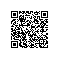# Python深入05 装饰器

### 装饰函数和方法

# get square sum
def square_sum(a, b):
return a**2 + b**2

# get square diff
def square_diff(a, b):
return a**2 - b**2
print(square_sum(3, 4))
print(square_diff(3, 4))

# modify: print input

# get square sum
def square_sum(a, b):
print("intput:", a, b)
return a**2 + b**2

# get square diff
def square_diff(a, b):
print("input", a, b)
return a**2 - b**2
print(square_sum(3, 4))
print(square_diff(3, 4))

def decorator(F):
def new_F(a, b):
print("input", a, b)
return F(a, b)
return new_F

# get square sum
@decorator
def square_sum(a, b):
return a**2 + b**2

# get square diff
@decorator
def square_diff(a, b):
return a**2 - b**2

print(square_sum(3, 4))
print(square_diff(3, 4))

square_sum = decorator(square_sum)
square_sum(3, 4)

### 含参的装饰器

# a new wrapper layer
def pre_str(pre=''):
# old decorator
def decorator(F):
def new_F(a, b):
print(pre + "input", a, b)
return F(a, b)
return new_F
return decorator

# get square sum
@pre_str('^_^')
def square_sum(a, b):
return a**2 + b**2

# get square diff
@pre_str('T_T')
def square_diff(a, b):
return a**2 - b**2

print(square_sum(3, 4))
print(square_diff(3, 4))

square_sum = pre_str('^_^') (square_sum)

### 装饰类

def decorator(aClass):
class newClass:
def __init__(self, age):
self.total_display   = 0
self.wrapped         = aClass(age)
def display(self):
self.total_display += 1
print("total display", self.total_display)
self.wrapped.display()
return newClass

@decorator
class Bird:
def __init__(self, age):
self.age = age
def display(self):
print("My age is",self.age)

eagleLord = Bird(5)
for i in range(3):
eagleLord.display()

### 总结使用钉钉扫一扫加入圈子
+ 订阅